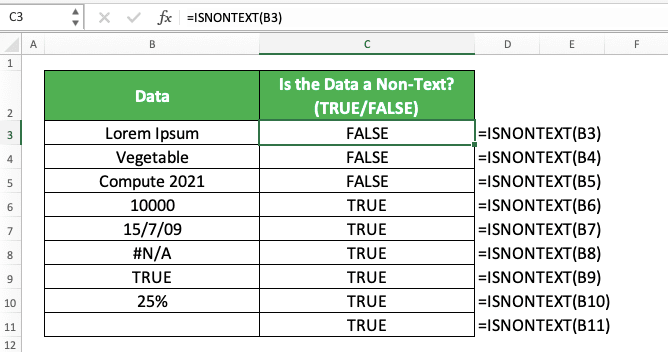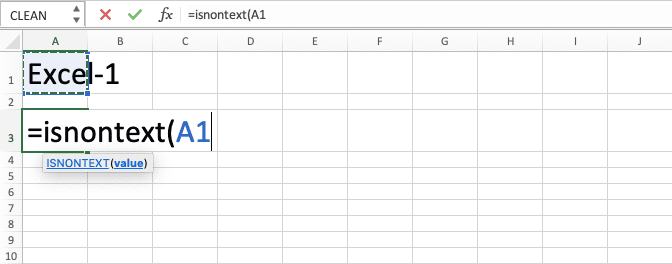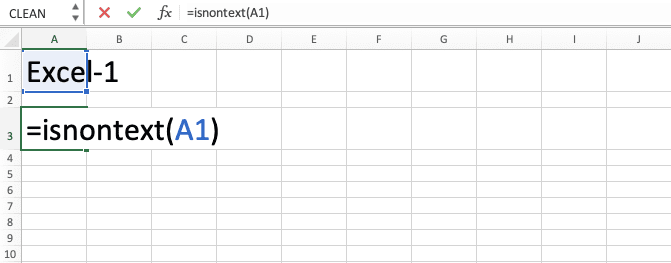How to Use the ISNONTEXT Function in Excel: Usabilities, Examples, and Writing Steps - Compute Expert

# How to Use the ISNONTEXT Function in Excel: Usabilities, Examples, and Writing Steps

Home >> Excel Tutorials from Compute Expert >> Excel Formulas List >> How to Use the ISNONTEXT Function in Excel: Usabilities, Examples, and Writing Steps

In this tutorial, you will learn how to use the ISNONTEXT function in excel completely.

When working with our data in excel, we sometimes need to identify whether our data is a non-text or not. If we use ISNONTEXT, then we can do the identification process fast and easily.

Want to know more about this ISNONTEXT function and how to use it properly in excel? Read this tutorial until its last part!

Disclaimer: This post may contain affiliate links from which we earn commission from qualifying purchases/actions at no additional cost for you. Learn more

## What is the ISNONTEXT Function in Excel?

ISNONTEXT is one of the IS functions in excel that helps you to identify whether a data is a non-text.

## ISNONTEXT Usability

You can use ISNONTEXT to identify whether your data is a non-text or not. You will get a TRUE or FALSE logic value based on the identification result.

## ISNONTEXT Result

The ISNONTEXT result is a logic value (TRUE or FALSE). The logic value you get depends on whether your data is a non-text (TRUE) or not (FALSE).

## Excel Version from Which We Can Start Using ISNONTEXT

We can start using ISNONTEXT in excel since excel 2003.

## The Way to Write It and Its Input

Here is the general writing form of ISNONTEXT in excel.

= ISNONTEXT ( data )

You can input the data you want to identify with ISNONTEXT directly or through a cell coordinate.

## Example of Its Usage and Result

Here is an implementation example of ISNONTEXT in excel.As you can see in the example, it is quite easy to implement ISNONTEXT in excel. Just input the data we want to identify, press enter, and we will immediately get its result!

You can also see in the example that ISNONTEXT only produces FALSE to text data. It produces TRUE for other data types such as number, logical value, empty cell, or even an error.

## Writing Steps

After we discussed the ISNONTEXT writing form, input, and implementation example, now let’s discuss its writing steps. As ISNONTEXT only needs the data you want to identify as its input, its writing steps should be easy to understand.

1. Type an equal sign ( = ) in the cell where you want to put the ISNONTEXT identification result2. Type ISNONTEXT (can be with large and small letters) and an open bracket sign after =3. Input the data you want to identify whether it is a non-text or not4. Type a close bracket sign5. Press Enter
6. Done!## Get a Particular Result if Our Data is a Non-Text: IF ISNONTEXT

Want to get a particular result after you get your TRUE or FALSE result from ISNONTEXT? You can combine it with an IF formula for that.

Here is the general writing form of the IF and ISNONTEXT formulas combination in excel.

= IF ( ISNONTEXT ( data ) , result_if_nontext , result_if_text )

Simple, right? We just write our ISNONTEXT in the logical condition input part of our IF.

We also input the IF result we want if ISNONTEXT produces a TRUE or FALSE. By doing that, we will get a particular result if our data is a non-text or if it isn’t!

Here is the implementation example of the two formulas combination in excel.As you can see, we can change the non-text/text identifier with something we want by combining IF and ISNONTEXT. In the example, we use identifiers that make the mark for the non-text/text data much clearer.

## Exercise

After you have understood how to use ISNONTEXT in excel, now let’s do an exercise so you can deepen your understanding.

### Questions

1. Is the cell content on the left non-text? Answer with TRUE or FALSE!
2. Is the cell content on the left non-text? Answer with “V” if yes and “X” if not!
3. Is the cell content on the left non-text? Sum it with 100 if it is true and convert it to 0 if false!

In excel, we often combine ISNONTEXT with IF as the ISNONTEXT result is a logical value (TRUE or FALSE). We usually don’t need a logical value as our final result. Thus, we combine the formula which produces it with another formula that uses a logical value input such as IF.

Related tutorials you should learn too:

Get updated excel info from Compute Expert by registering your email. It's free!